# Pictographs#### All in One Place

Everything you need for JC, LC, and college level maths and science classes.#### Learn with Ease

We’ve mastered the national curriculum so that you can revise with confidence.#### Instant Help

0/4
##### Intros
###### Lessons
1. Introduction to Pictographs:
2. What is a pictograph?
3. How to read a pictograph
4. Understanding symbols and half symbol scales
5. Choosing a scale for your data
0/16
##### Examples
###### Lessons
1. Understanding symbols and scale
Fill in the blanks about the symbols and scale
1. If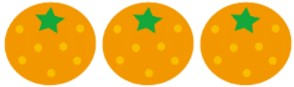represents 6 oranges, then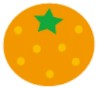represents ___ oranges.
2. If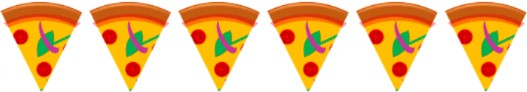represents 60 pizza slices, thenrepresents ___ pizza slices.
3. If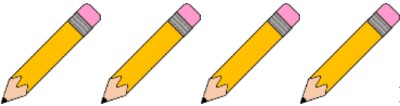represents ___ pencils, then represents 5 pencils.
4. If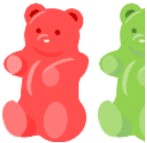represents 6 gummy bears, then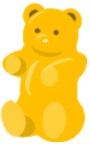represents ___ gummy bears.
5. If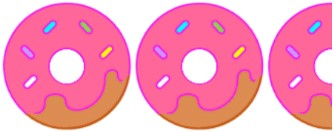represents ___ donuts, then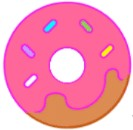represents 6 donuts.
2. Reading and understanding data displayed on a pictograph
Use the pictograph to answer the questions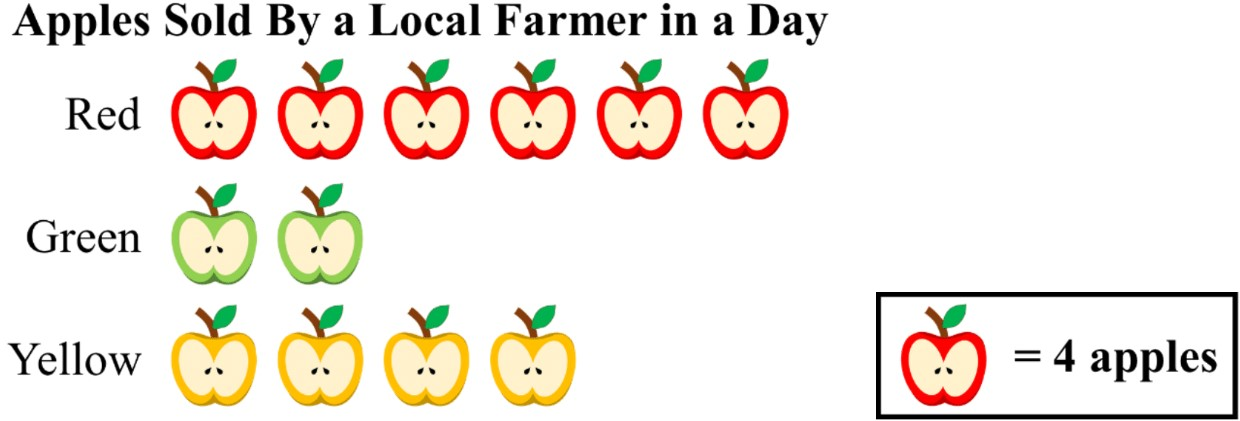1. How many of each type of apple was sold?
2. How many apples were sold in total?
3. Redraw the pictograph with a different (bigger) scale.
3. Reading and understanding data displayed on a pictograph (with half symbols)
Use the pictograph to answer the questions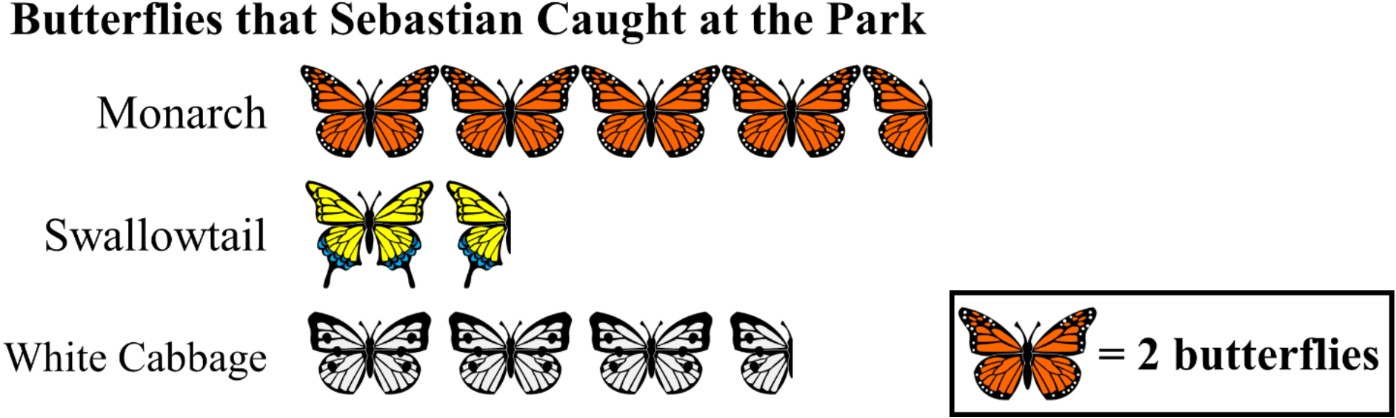1. Fill in the table for the number represented by the symbols.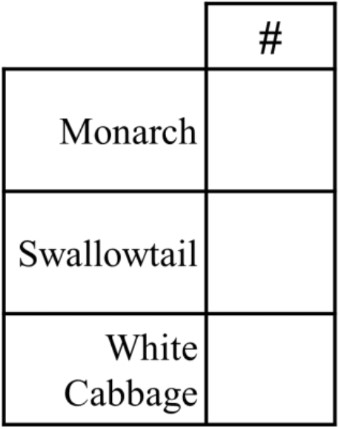2. How many butterflies did he catch in total?
3. How many times as many monarchs did he catch as swallowtails?
4. How many more white cabbages did he catch than swallowtails?
4. Creating a pictograph
Answer the questions for creating a pictograph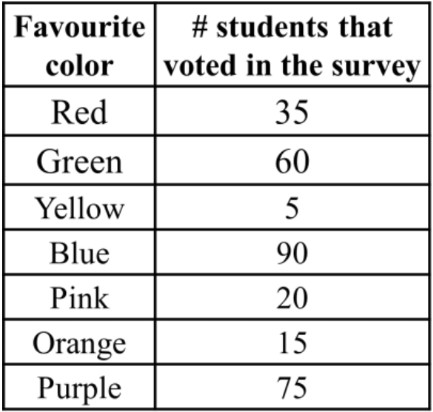1. Look at the table of data. What is the scale for the pictograph? The symbol of a circle will be used.
2. Draw in the symbols for the pictograph to display the data.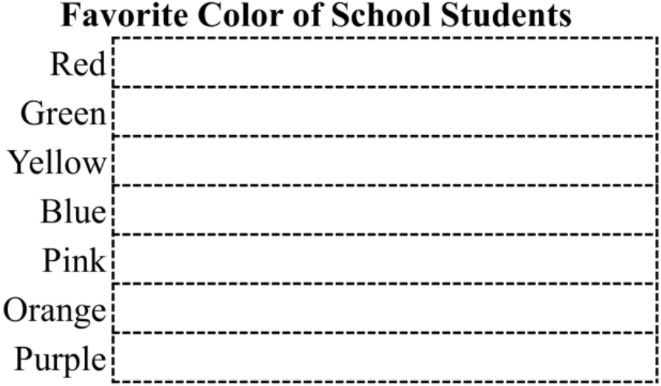3. Order the most common favorite color to the least favorite.
4. If there are actually 321 students, did everyone take the survey?
###### Topic Notes

In this lesson, we will learn:

• How to read a pictograph
• How to understand the symbols and scales for pictographs
• How to choose a scale when creating a pictograph

Notes:

• A pictograph is a graph that displays frequency data using picture symbols
• It is a great visual tool to show numbers in different categories from data collection experiments (i.e. surveys)

• The parts of a pictograph can include:
• a title to describe the data collected
• categories listed vertically (and sometimes, the category column names)
• (sometimes) a vertical label to describe the types of categories
• a key to show the symbol and scale (how much each symbol is worth)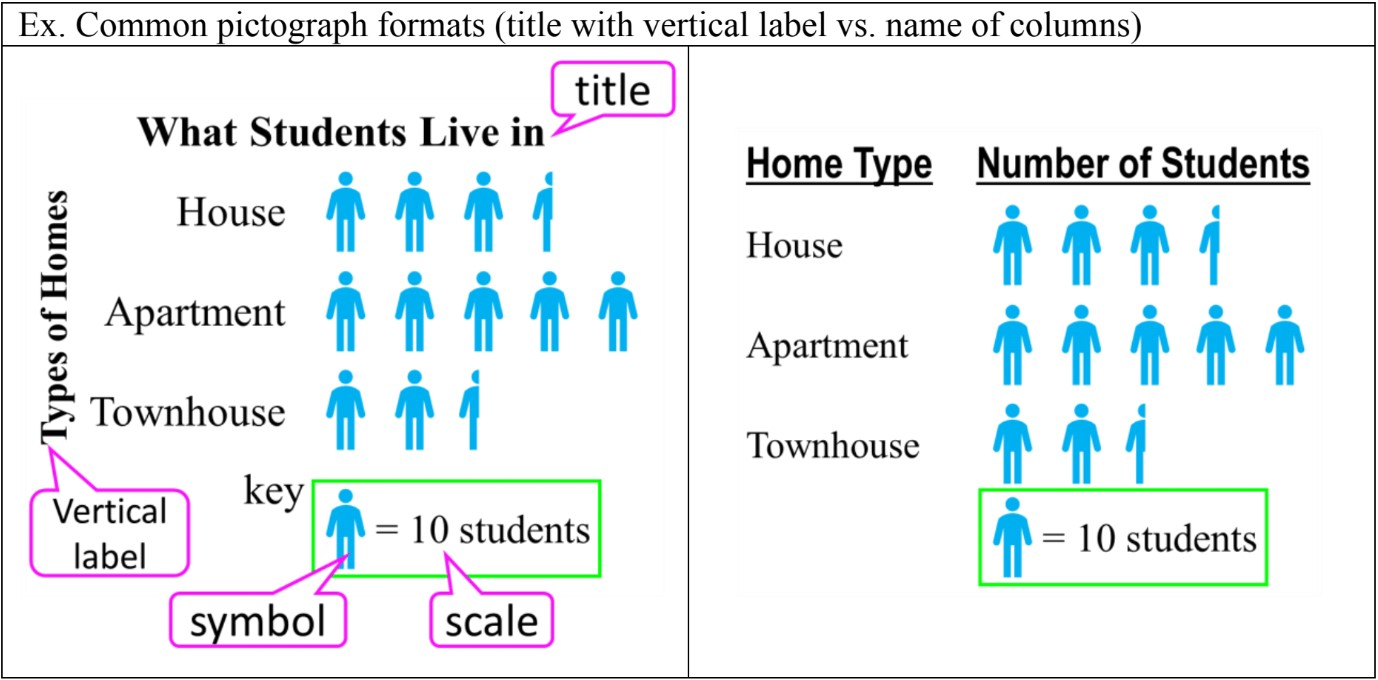• Symbol scales can use whole symbols, half symbols, or even fractional symbols: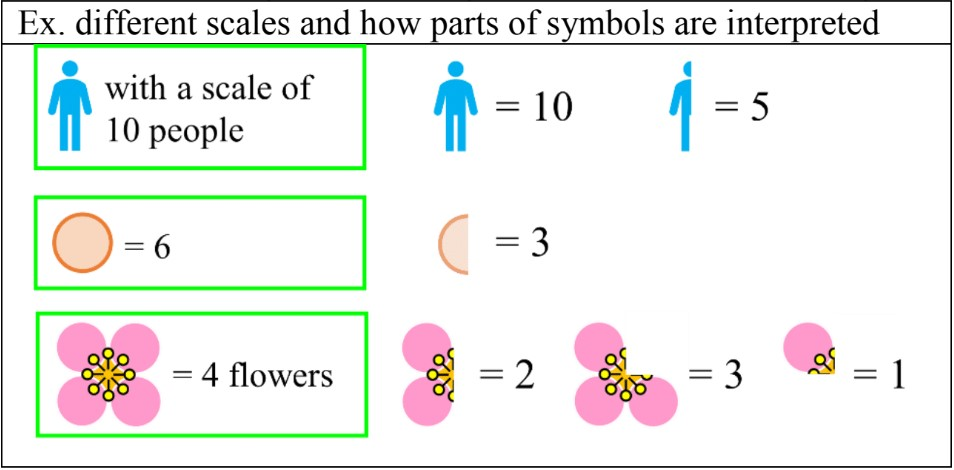• To choose a scale for a data set, find a common factor for all the numbers (a number that can be multiplied into all the numbers of the data set). It is that more than one scale will work.
• Ex. a data set with a scale of 4 could also use a scale of 8 (doubled); the pictograph will look neater and use less symbols to represent numbers